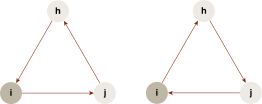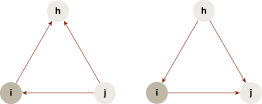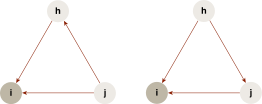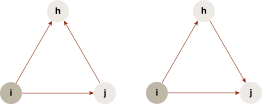# Unweighted, Directed Network (BDN) - Teradata Vantage

## Teradata® Vantage Machine Learning Engine Analytic Function Reference

Product
Release Number
1.0
8.00
Published
May 2019
Language
English (United States)
Last Update
2019-11-22
dita:mapPath
blj1506016597986.ditamap
dita:ditavalPath
blj1506016597986.ditaval
dita:id
lmf1502735330121
Product Category

For an unweighted, directed network (BDN), given a vertex i, the BDN triangle types can be categorized to four patterns.

Triangle Type Patterns
Pattern Description Illustration
Cycle There is a cyclical relation among i and any two of its neighbors: ijhi, or the reverse.Middleman One of i’s neighbors, j, both holds an outward edge to a third neighbor, h, and uses i to reach h in two steps.In i holds two inward edges.Out i holds two outward edges.For each pattern, this is the formula for the clustering coefficient (CC):

c i * = δ i * / τ i *

where {*}={cycle, middleman, in, out}.

These are the triples for each pattern:

τ i cyc = d i in d i out - d i

where d i is the number of bilateral edges between i and its neighbors.

τ i mid = d i in d i out - d i

τ i in = d i in (d i in - 1)

τ i out = d i out (d i out - 1)